# Algorithms for Advanced Hyper-Parameter Optimization/Tuning

In informed search, each iteration learns from the last, whereas in Grid and Random, modelling is all done at once and then the best is picked. In case for small datasets, GridSearch or RandomSearch would be fast and sufficient. AutoML approaches provide a neat solution to properly select the required hyperparameters that improve the model’s performance.

By Gowrisankar JG, Software Developer at Hexaware

Most Professional Machine Learning practitioners follow the ML Pipeline as a standard, to keep their work efficient and to keep the flow of work. A pipeline is created to allow data flow from its raw format to some useful information. All sub-fields in this pipeline’s modules are equally important for us to produce quality results, and one of them is Hyper-Parameter Tuning.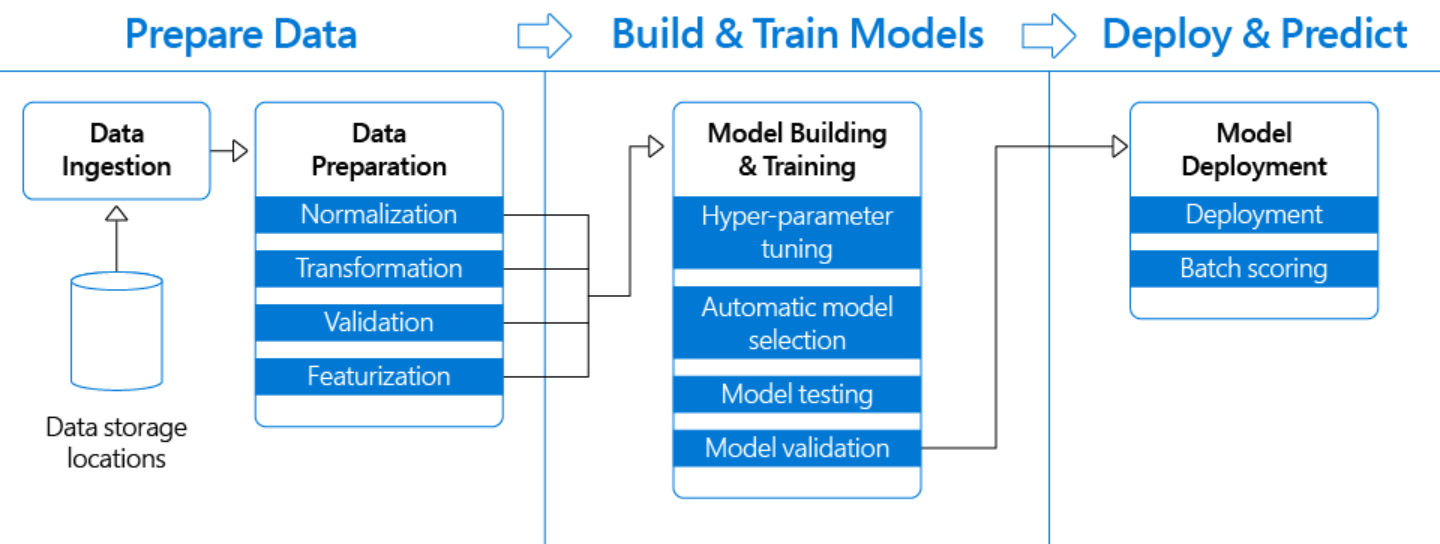A Generalized Machine Learning Pipeline

Most of us know the best way to proceed with Hyper-Parameter Tuning is to use the GridSearchCV or RandomSearchCV from the sklearn module. But apart from these algorithms, there are many other Advanced methods for Hyper-Parameter Tuning. This is what the article is all about, Introduction to Advanced Hyper-Parameter Optimization, Transfer Learning and when & how to use these algorithms to make out the best of them.

Both of the algorithms, Grid-Search and Random-Search are instances of Uninformed Search. Now, let’s dive deep !!

### Uninformed search

Here in these algorithms, each iteration of the Hyper-parameter tuning does not learn from the previous iterations. This is what allows us to parallelize our work. But, this isn’t very efficient and costs a lot of computational power.

Random search tries out a bunch of hyperparameters from a uniform distribution randomly over the preset list/hyperparameter search space (the number iterations is defined). It is good in testing a wide range of values and normally reaches to a very good combination very fastly, but the problem is that, it doesn’t guarantee to give the best parameter’s combination.

On the other hand, Grid search will give the best combination, but it can takes a lot of time and the computational cost is high.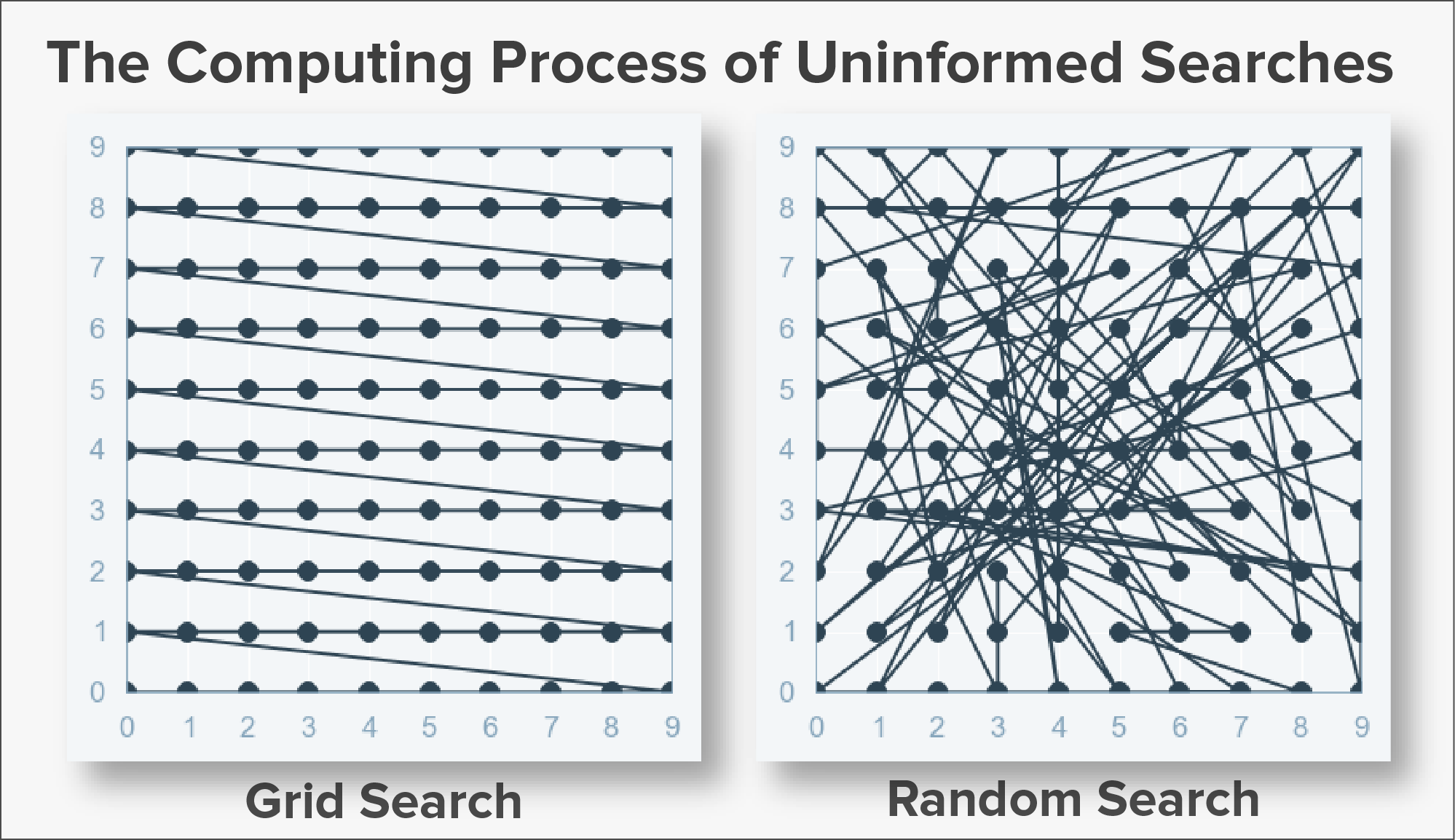Searching Pattern of Grid and Random Search

It may look like grid search is the better option, compared to the random one, but bare in mind that when the dimensionality is high, the number of combinations we have to search is enormous. For example, to grid-search ten boolean (yes/no) parameters you will have to test 1024 (2¹⁰) different combinations. This is the reason, why random search is sometimes combined with clever heuristics, is often used.

### Why bring Randomness in Grid Search? [Mathematical Explanation]

Random search is more of a stochastic search/optimization perspective — the reason we introduce noise (or some form of stochasticity) into the process is to potentially bounce out of poor local minima. While this is more typically used to explain the intuition in general optimization (like stochastic gradient descent for updating parameters, or learning temperature-based models), we can think of humans looking through the meta-parameter space as simply a higher-level optimization problem. Since most would agree that these dimensional spaces (reasonably high) lead to a non-convex form of optimization, we humans, armed even with some clever heuristics from the previous research, can get stuck in the local optima.

Therefore, Randomly exploring the search space might give us better coverage, and more importantly, it might help us find better local optima.

Sofar in Grid and Random Search Algorithms, we have been creating all the models at once and combining their scores before deciding the best model at the end.

An alternative approach would be to build models sequentially, learning from each iteration. This approach is termed as Informed Search.

### Informed Method: Coarse to Fine Tuning

A basic informed search methodology.

The process follows:

1. Random search
2. Find promising areas in the search space
3. Grid search in the smaller area
4. Continue until optimal score is obtained

You could substitute (3) with random searches before the grid search.

### Why Coarse to Fine?

Coarse to Fine tuning optimizes and uses the advantages of both grid and random search.

• Wide searching capabilities of random search
• Deeper search once you know where a good spot is likely to be

No need to waste time on search spaces that are not giving good results !! Therefore, this better utilizes the spending of time and computational efforts, i.e we can iterate quickly, also there is boost in the performance.

### Informed Method: Bayesian Statistics

The most popular informed search method is Bayesian Optimization. Bayesian Optimization was originally designed to optimize black-box functions.

This is a basic theorem or rule from Probability Theory and Statistics, in case if you want to brush up and get refreshed with the terms used here, refer this.

Bayes Rule | Theorem
A statistical method of using new evidence to iteratively update our beliefs about some outcome. In simpler words, it is used to calculate the probability of an event based on its association with another event.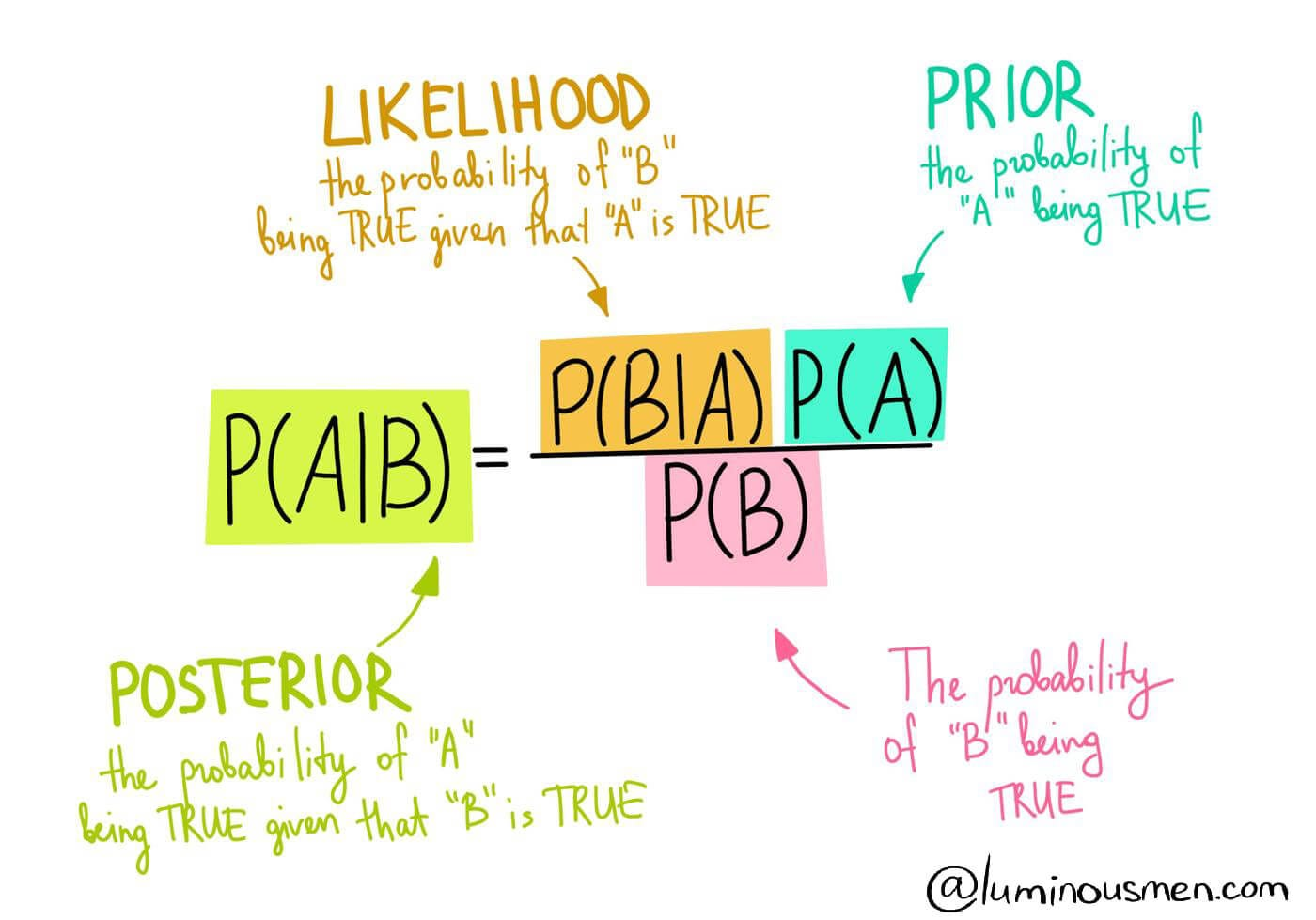• LHS is the probability of A, given B has occurred. B is some new evidence. This is known as the ‘posterior’.
• RHS is how we calculate this.
• P(A) is the ‘prior’. The initial hypothesis about the event. It is different to P(A|B), the P(A|B) is the probability given new evidence.
• P(B) is the ‘marginal likelihood’ and it is the probability of observing this new evidence.
• P(B|A) is the ‘likelihood’ which is the probability of observing the evidence, given the event we care about.

Applying the logic of Bayes rule to hyperparameter tuning:

1. Pick a hyperparameter combination
2. Build a model
3. Get new evidence (i.e the score of the model)
4. Update our beliefs and chose better hyperparameters next round

Bayesian hyperparameter tuning is quite new but is very popular for larger and more complex hyperparameter tuning tasks as they work well to find optimal hyperparameter combinations in these kinds of situations.

### Note

For more complex cases you might want to dig a bit deeper and explore all the details about Bayesian optimization. Bayesian optimization can only work on continuous hyper-parameters, and not categorical ones.

### Bayesian Hyper-parameter Tuning with HyperOpt

HyperOpt package, uses a form of Bayesian optimization for parameter tuning that allows us to get the best parameters for a given model. It can optimize a model with hundreds of parameters on a very large scale.To know more about this library and the parameters of HyperOpt library feel free to visit here. And visit here for a quick tutorial with adequate explanation on how to use HyperOpt for Regression and Classification.

### Introducing the HyperOpt package.

To undertake Bayesian hyperparameter tuning we need to:

1. Set the Domain: Our Grid i.e. search space (with a bit of a twist)
2. Set the Optimization algorithm (default: TPE)
3. Objective function to minimize: we use “1-Accuracy”

Know more about the Optimization Algorithm used, Original Paper of TPE (Tree of Parzen Estimators)

### Sample Code for using HyperOpt [ Random Forest ]

HyperOpt does not use point values on the grid but instead, each point represents probabilities for each hyperparameter value. Here, simple uniform distribution is used, but there are many more if you check the documentation.

HyperOpt implemented on Random Forest

To really see this in action !! try on a larger search space, with more trials, more CVs and a larger dataset size.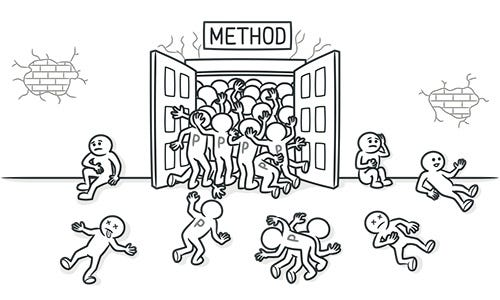### For practical implementation of HyperOpt refer:

Curious to know why XGBoost has high potential in winning competitions ?? Read the below article to expand your knowledge !!

XGBoost — Queen of Boosting Algorithms?
Kaggler’s Favo Algorithm | Understanding How & Why XGBoost is used to win Kaggle competitions

### Informed Method: Genetic Algorithms

Why does this work well?

1. It allows us to learn from previous iterations, just like Bayesian hyperparameter tuning.
3. TPOT will automate the most tedious part of machine learning by intelligently exploring thousands of possible pipelines to find the best one for your data.

### A useful library for genetic hyperparameter tuning: TPOT

TPOT is a Python Automated Machine Learning tool that optimizes machine learning pipelines using genetic programming.

Consider TPOT your Data Science Assistant for advanced optimization.

Pipelines not only include the model (or multiple models) but also work on features and other aspects of the process. Plus it returns the Python code of the pipeline for you! TPOT is designed to run for many hours to find the best model. You should have a much larger population and offspring size as well as hundreds of more generations to find a good model.

### TPOT Components ( Key Arguments )

• generations — Iterations to run training for
• population_size — The number of models to keep after each iteration
• offspring_size — Number of models to produce in each iteration
• mutation_rate — The proportion of pipelines to apply randomness to
• crossover_rate — The proportion of pipelines to breed each iteration
• scoring — The function to determine the best models
• cv — Cross-validation strategy to use

TPOT Classifier

We will keep default values for mutation_rate and crossover_rate as they are best left to the default without deeper knowledge on genetic programming.

### Notice: No algorithm-specific hyperparameters?

Since TPOT is an open-source library for performing AutoML in Python.

AutoML ??

Automated Machine Learning (AutoML) refers to techniques for automatically discovering well-performing models for predictive modeling tasks with very little user involvement.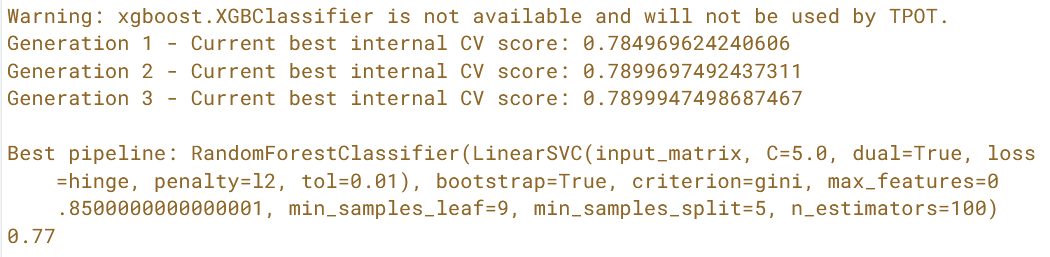Output for the above code snippet

TPOT is quite unstable when not run for a reasonable amount of time. The below code snippets shows the instability of TPOT. Here, only the random state has been changed in the below three codes, but the Output shows major differences in choosing the pipeline, i.e. model and it’s hyperparameters.

You can see in the output the score produced by the chosen model (in this case a version of Naive Bayes) over each generation, and then the final accuracy score with the hyperparameters chosen for the final model. This is a great first example of using TPOT for automated hyperparameter tuning. You can now extend this on your own and build great machine learning models!

 TPOT for Automated Machine Learning in Python

### Summary

In informed search, each iteration learns from the last, whereas in Grid and Random, modelling is all done at once and then the best is picked. In case for small datasets, GridSearch or RandomSearch would be fast and sufficient.

AutoML approaches provide a neat solution to properly select the required hyperparameters that improve the model’s performance.

Informed methods explored were:

1. ‘Coarse to Fine’ (Iterative random then grid search).
2. Bayesian hyperparameter tuning, updating beliefs using evidence on model performance (HyperOpt).
3. Genetic algorithms, evolving your models over generations (TPOT).

I hope you’ve learned some useful methodologies for your future work undertaking hyperparameter tuning in Python!

Create REST API in Minutes With Go / Golang
In this article, there is a short comparison between different routers and then the walk-through to create a REST API…

If you are curious to know about Golang’s Routers and want to try out a simple web development project using Go, I suggest to read the above article.References

Bio: Gowrisankar JG (@jg_gowrisankar) is passionate about Data Science, Data Analytics, Machine Learning and #NLP, and is a Software Developer at Hexaware.

Original. Reposted with permission.

Related: